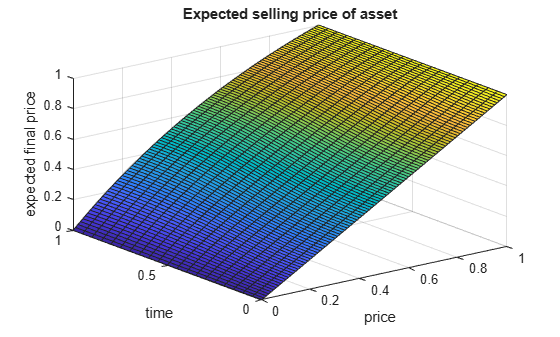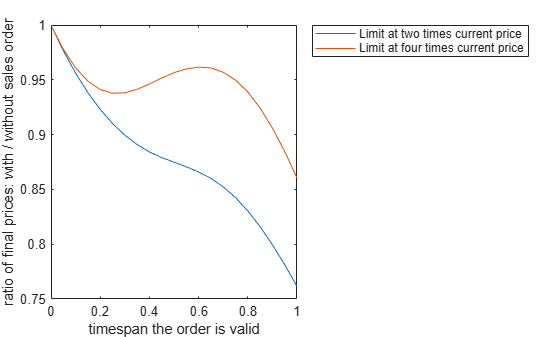# Simulate a Stochastic Process Using the Feynman–Kac Formula

This example obtains the partial differential equation that describes the expected final price of an asset whose price is a stochastic process given by a stochastic differential equation.

The steps involved are:

1. Define Parameters of the Model Using Stochastic Differential Equations

2. Apply Ito's Rule

3. Solve Feynman–Kac Equation

4. Compute Expected Time to Sell Asset

### 1. Define Parameters of the Model Using Stochastic Differential Equations

A model for the price of an asset `X(t)` defined in the time interval `[0,T]` is a stochastic process defined by a stochastic differential equation of the form $dX=\mu \left(t,X\right)dt+\sigma \left(t,X\right)dB\left(t\right)$, where `B(t)` is the Wiener process with unit variance parameter.

Create the symbolic variable `T` and the following symbolic functions:

• `r(t)` is a continuous function representing a spot interest rate. This rate determines the discount factor for the final payoff at the time `T`.

• `u(t,x)` is the expected value of the discounted future price calculated as $\mathit{X}\left(\mathit{T}\right)\text{\hspace{0.17em}}\mathrm{exp}\left(-{\int }_{\mathit{t}}^{\mathit{T}}\mathit{r}\left(\mathit{t}\right)\text{\hspace{0.17em}}\mathrm{dt}\right)$ under the condition `X(t) = x`.

• $\mu \left(t,X\right)$ and $\sigma \left(t,X\right)$ are drift and diffusion of the stochastic process `X(t)`.

`syms mu(t, X) sigma(t, X) r(t, X) u(t, X) T`

According to the Feynman–Kac theorem, `u` satisfies the partial differential equation $\frac{\partial u}{\partial t}+\frac{{\sigma }^{2}}{2}\frac{{\partial }^{2}u}{\partial {x}^{2}}+\mu \frac{\partial u}{\partial x}-ur=0$ with a final condition at time `T`.

`eq = diff(u, t) + sigma^2*diff(u, X, X)/2 + mu*diff(u, X) - u*r;`

The numerical solver `pdepe` works with initial conditions. To transform the final condition into an initial condition, apply a time reversal by setting `u(t, X) = v(T - t, X)`.

```syms v(t, X) eq2 = subs(eq, {u, t}, {v(T - t, X), T - t}); eq2 = feval(symengine, 'rewrite', eq2, 'diff')```
```eq2 =  ```

The solver `pdepe` requires the partial differential equation in the following form. Here the coefficients `c`, `f`, and `s` are functions of `x`, `t`, `v`, and $\partial v/\partial x$.

`$c\frac{\partial v}{\partial t}=\frac{\partial f}{\partial x}+s$`

To be able to solve the equation `eq2` with the `pdepe` solver, map `eq2` to this form. First, extract the coefficients and terms of `eq2`.

```syms dvt dvXX eq3 = subs(eq2, {diff(v, t), diff(v, X, X)}, {dvt, dvXX}); [k,terms] = coeffs(eq3, [dvt, dvXX])```
```k =  ```
`terms = $\left(\begin{array}{ccc}\mathrm{dvt}& \mathrm{dvXX}& 1\end{array}\right)$`

Now, you can rewrite `eq2` as `k(1)*terms(1) + k(2)*terms(2) + k(3)*terms(3) = 0`. Moving the term with the time derivative to the left side of the equation, you get

`$\frac{\partial v}{\partial t}=k\left(2\right)\frac{{\partial }^{2}v}{\partial {X}^{2}}+k\left(3\right)$`

Manually integrate the right side by parts. The result is

`$\frac{\partial }{\partial X}\left(k\left(2\right)\frac{\partial v}{\partial X}\right)+k\left(3\right)-\frac{\partial v}{\partial X}\frac{\partial k\left(2\right)}{\partial X}$`

Therefore, to write `eq2` in the form suitable for `pdepe`, use the following parameters:

```c = 1; f = k(2) * diff(v, X); s = k(3) - diff(v, X) * diff(k(2), X);```

### 2. Apply Ito's Rule

Asset prices follow a multiplicative process. That is, the logarithm of the price can be described in terms of an SDE, but the expected value of the price itself is of interest because it describes the profit, and thus we need an SDE for the latter.

In general, if a stochastic process `X` is given in terms of an SDE, then Ito's rule says that the transformed process `G(t, X)` satisfies

`$dG=\left(\mu \frac{dG}{dX}+\frac{{\sigma }^{2}}{2}\frac{{d}^{2}G}{d{X}^{2}}+\frac{dG}{dt}\right)dt+\frac{dG}{dX}\sigma dB\left(t\right)$`

We assume that the logarithm of the price is given by a one-dimensional additive Brownian motion, that is, `mu` and `sigma` are constants. Define a function that applies Ito's rule, and use it to transform the additive Brownian motion into a geometric Brownian motion.

```ito = @(mu, sigma, G, t, X) ... deal( mu * diff(G, X) + sigma^2/2 * diff(G, X, X) + diff(G, t), ... diff(G, X) * sigma ); syms mu0 sigma0 [mu1, sigma1] = ito(mu0, sigma0, exp(X), t, X)```
```mu1 =  $\frac{{\mathrm{e}}^{X} {{\sigma }_{0}}^{2}}{2}+{\mu }_{0} {\mathrm{e}}^{X}$```
`sigma1 = ${\sigma }_{0} {\mathrm{e}}^{X}$`

Replace `exp(X)` by `Y`.

```syms Y mu1 = subs(mu1, X, log(Y)); sigma1 = subs(sigma1, X, log(Y)); f = f(t, log(Y)); s = s(t, log(Y));```

For simplicity, assume that the interest rate is zero. This is a special case also known as Kolmogorov backward equation.

```r0 = 0; c = subs(c, {mu, sigma}, {mu1, sigma1}); s = subs(s, {mu, sigma, r}, {mu1, sigma1, r0}); f = subs(f, {mu, sigma}, {mu1, sigma1});```

### 3. Solve Feynman–Kac Equation

Before you can convert symbolic expressions to MATLAB function handles, you must replace function calls, such as `diff(v(t, X), X)` and `v(t, X)`, with variables. You can use any valid MATLAB variable names.

```syms dvdx V; dvX = diff(v, X); c = subs(c, {dvX, v}, {dvdx, V}); f = subs(f, {dvX, v}, {dvdx, V}); s = subs(s, {dvX, v}, {dvdx, V});```

For a flat geometry (translation symmetry), set the following value:

`m = 0;`

Also, assign numeric values to symbolic parameters.

```muvalue = 0; sigmavalue = 1; c0 = subs(c, {mu0, sigma0}, {muvalue, sigmavalue}); f0 = subs(f, {mu0, sigma0}, {muvalue, sigmavalue}); s0 = subs(s, {mu0, sigma0}, {muvalue, sigmavalue});```

Use `matlabFunction` to create a function handle. Pass the coefficients `c0`, `f0`, and `s0` in the form required by `pdepe`, namely, a function handle with three output arguments.

`FeynmanKacPde = matlabFunction(c0, f0, s0, 'Vars', [t, Y, V, dvdx])`
```FeynmanKacPde = function_handle with value: @(t,Y,V,dvdx)deal(1.0,(Y.^2.*dvdx)./2.0,(Y.*dvdx)./2.0) ```

As the final condition, take the identity mapping. That is, the payoff at time $T$ is given by the asset price itself. You can modify this line in order to investigate derivative instruments.

`FeynmanKacIC = @(x) x;`

Numerical solving of PDEs can only be applied to a finite domain. Therefore, you must specify a boundary condition. Assume that the asset is sold at the moment when its price rises above or falls below a certain limit, and thus the solution `v` has to satisfy `x - v = 0` at the boundary points `x`. You can choose another boundary condition, for example, you can use `v = 0` to model knockout options. The zeroes in the second and fourth output indicate that the boundary condition does not depend on $\frac{dv}{dx}$.

```FeynmanKacBC = @(xl, vl, xr, vr, t) ... deal(xl - vl, 0, xr - vr, 0);```

Choose the space grid, which is the range of values of the price `x`. Set the left boundary to zero: it is not reachable by a geometric random walk. Set the right boundary to one: when the asset price reaches this limit, the asset is sold.

`xgrid = linspace(0, 1, 101);`

Choose the time grid. Because of the time reversal applied in the beginning, it denotes the time left until the final time `T`.

`tgrid = linspace(0, 1, 21);`

Solve the equation.

`sol = pdepe(m,FeynmanKacPde,FeynmanKacIC,FeynmanKacBC,xgrid,tgrid);`

Plot the solution. The expected selling price depends nearly linearly on the price at time `t`, and also weakly on `t`.

```surf(xgrid, tgrid, sol) title('Expected selling price of asset'); xlabel('price'); ylabel('time'); zlabel('expected final price');```The state of a geometric Brownian motion with drift $\mu 1$ at time `t` is a lognormally distributed random variable with expected value $\mathrm{exp}\left({\mu }_{1}t\right)$ times its initial value. This describes the expected selling price of an asset that is never sold because of reaching a limit.

`Expected = transpose(exp(tgrid./2)) * xgrid;`

Dividing the solution obtained above by that expected value shows how much profit is lost by selling prematurely at the limit.

```sol2 = sol./Expected; surf(xgrid, tgrid, sol2) title('Ratio of expected final prices: with / without sales order at x=1') xlabel('price'); ylabel('time'); zlabel('ratio of final prices');```For example, plot the ratio of the expected payoff of an asset for which a limit sales order has been placed and the same asset without sales order over a timespan `T`, as a function of `t`. Consider the case of an order limit of two and four times the current price, respectively.

```plot(tgrid, sol2(:, xgrid == 1/2)); hold on plot(tgrid, sol2(:, xgrid == 1/4)); legend('Limit at two times current price', 'Limit at four times current price'); xlabel('timespan the order is valid'); ylabel('ratio of final prices: with / without sales order'); hold off```### 4. Compute Expected Time to Sell Asset

It is a textbook result that the expected exit time when the limit is reached and the asset is sold is given by the following equation:

```syms y(X) exitTimeEquation(X) = subs(eq, {r, u}, {0, y(X)}) == -1```
```exitTimeEquation(X) =  ```

In addition, `y` must be zero at the boundaries. For `mu` and `sigma`, insert the actual stochastic process under consideration:

`exitTimeGBM = subs(subs(exitTimeEquation, {mu, sigma}, {mu1, sigma1}), Y, X)`
```exitTimeGBM(X) =  ```

Solve this problem for arbitrary interval borders `a` and `b`.

```syms a b exitTime = dsolve(exitTimeGBM, y(a) == 0, y(b) == 0)```
```exitTime =  ```

Because you cannot substitute `mu0 = 0` directly, compute the limit at `mu0 -> 0` instead.

`L = limit(subs(exitTime, sigma0, sigmavalue), mu0, muvalue)`
`L = $-\left(\mathrm{log}\left(X\right)-\mathrm{log}\left(a\right)\right) \left(\mathrm{log}\left(X\right)-\mathrm{log}\left(b\right)\right)$`

`L` is undefined at `a = 0`. Set the assumption that `0 < X < 1`.

`assume(0 < X & X < 1)`

Using the value `b = 1` for the right border, compute the limit.

`limit(subs(L, b, 1), a, 0, 'Right')`
`ans = $\infty$`

The expected exit time is infinite.